# ECMA-262-5 详解 - 3.1 词法环境：通用理论 – ds.laboratory

2018年11月03日 发布 阅读(2212) 作者：Jerman

## 介绍

ECMA-262-5 规范初次引入了词法环境，尽管这一术语独立于 ECMAScript，在很多编程语言中被使用。

## 通用理论

### 作用域

``// 全局 "x"``int x = 10;``void foo() {``  // "foo" 函数的局部 "x"``  int x = 20;``  if (true) {``    // if 语句块的局部 "x"``    int x = 30;``    printf("%d", x); // 30``  }``  printf("%d", x); // 20``}``foo();``printf("%d", x); // 10``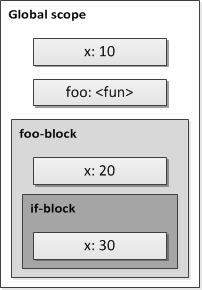ECMAScript 在版本 6 （也叫 ES6 或 ES2015）之前并不支持块级作用域：

``var x = 10;``if (true) {``  var x = 20;``  console.log(x); // 20``}``console.log(x); // 20``

ES6 规定 `let` 关键字用于创建块级作用域变量：

``let x = 10;``if (true) {``  let x = 20;``  console.log(x); // 20``}``console.log(x); // 10``

``var x = 10;``if (true) {``  (function (x) {``    console.log(x); // 20``  })(20);``}``console.log(x); // 10``

#### 静态（词法）作用域

“静态”指可以在程序的解析过程就决定作用域。这是指，如果我们（通过解析代码）在启动程序之前就能确定变量对应的作用域，那么我们是使用了静态作用域的方式。

``var x = 10;``var y = 20;``function foo() {``  console.log(x, y);``}``foo(); // 10, 20``function bar() {``  var y = 30;``  console.log(x, y); // 10, 30``  foo(); // 10, 20``}``bar();``

#### 动态作用域

``// 伪代码 - 使用动态作用域``y = 20;``procedure foo()``  print(y)``end``// 在栈上的名称 "y"``// 目前对于的值是 20``// {y: }``foo() // 20, OK``procedure bar()``  // 现在的栈上，有两个 "y" 的值：``  // {y: [20, 30]}``  // 取第一个值（从栈顶）``  y = 30``  // 所以：``  foo() // 30！不是 20``end``bar()``

``# Perl 示例：静态和动态作用域``\$a = 0;``sub foo {``  return \$a;``}``sub staticScope {``  my \$a = 1; # 词法（静态）``  return foo();``}``print staticScope(); # 0 （来自保存的全局帧）``\$b = 0;``sub bar {``  return \$b;``}``sub dynamicScope {``  local \$b = 1; # 动态``  return bar();``}``print dynamicScope(); # 1 （来自调用方的帧）``
##### ECMAScript 中 with 和 eval 的动态作用域特性

``var x = 10;``var o = {x: 30};``var storage = {};``(function foo(flag) {``  if (flag == 2) {``    eval("var x = 20;");``  }``  if (flag == 3) {``    storage = o;``  }``  with (storage) {``    // "x" 可以在全局作用域中求值 - 10，``    // 也可以在函数局部作用域中求值 - 20（通过"eval"函数），``    // 甚至在 "storage"对象中求值 - 30``    alert(x); // ? - "x" 的作用域在编译时决定``  }``  // 递归调用 3 次``  if (flag < 3) {``    foo(++flag);``  }``})(1);``

### 名称绑定

#### 重绑定

``// 将 "foo" 绑定到对象 {x: 10}``var foo = {x: 10};``console.log(foo.x); // 10``// 绑定 "bar" 到相同的对象``// 和标志符 "foo" 绑定对象相同``var bar = foo;``console.log(foo === bar); // true``console.log(bar.x); // OK，也是 10``// 重新绑定 "foo" 到新对象``foo = {x: 20};``console.log(foo.x); // 20``// "bar" 仍旧指向老对象``console.log(bar.x); // 10``console.log(foo === bar); // false``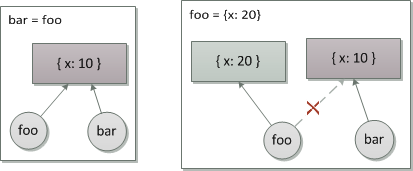#### 修改

``// 将一个数组绑定到标志符 "foo"``var foo = [1, 2, 3];``// 这是对数组对象的修改``foo.push(4);``console.log(foo); // 1,2,3,4``// 继续修改``foo = 5;``foo = 0;``console.log(foo); // 0,2,3,4,5``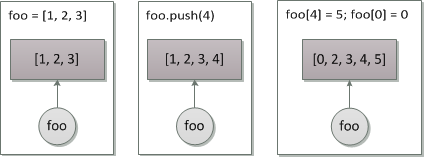### 环境

ECMAScript 通过使用调用栈模型来管理函数的执行，这里称之为 执行上下文栈。我们来看一些基本的存储变量（绑定）的模型。有闭包和没闭包的系统。

#### 激活记录模型

``void foo(int x) {``  int y = 20;``  bar(30);``}``void bar(x) {``  int z = 40;``}``foo(10);``

``callStack = [];``// "foo" 函数激活记录入栈``callStack.push({``  x: 10,``  y: 20``});``// "bar" 函数激活记录入栈``callStack.push({``  x: 30,``  z: 40``});``// "bar" 函数激活时的调用栈``console.log(callStack); // [{x: 10, y: 20}, {x: 30, z: 40}]``// "bar" 函数执行结束``callStack.pop();``// "foo" 函数执行结束``callStack.pop();``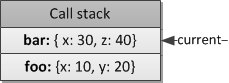ECMAScript 中也使用了相同的函数执行过程。不过，有些非常重要的不同。

``var bar = (function foo() {``  var x = 10;``  var y = 20;``  return function bar() {``    return x + y;``  };``})();``bar(); // 30``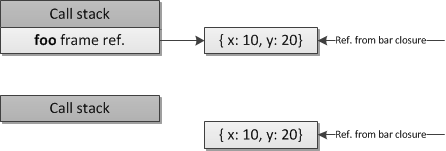#### 环境帧模型

##### 一等函数

``// 在运行时，动态创建一个函数表达式``// 绑定到标志符 "foo"``var foo = function () {``  console.log("foo");``};``// 将其传递给另一个函数，也是在运行时创建并``// 在创建后立即被调用，然后传入的函数并绑定``// 到标志符 "foo"``foo = (function (funArg) {``  // 激活 "foo" 函数``  funArg(); // "foo"``  // 作为值返回``  return funArg;``})(foo);``

##### 自由变量

``// 全局环境那（GE）``var x = 10;``function foo(y) {``  // 函数 "foo" 的环境（E1）``  var z = 30;``  function bar(q) {``    // 函数 "bar" 的环境（E2）``    return x + y + z + q;``  }``  // 返回 "bar" 到外部``  return bar;``}``var bar = foo(20);``bar(40); // 100``

##### 环境定义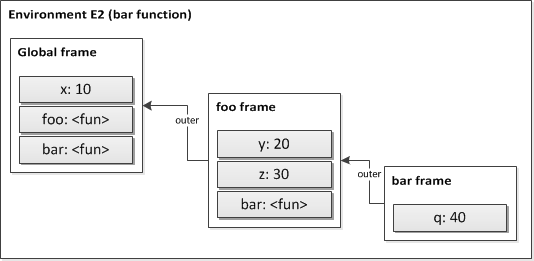``var x = 10;``(function foo(y) {``  // z使用自由绑定的变量 "x"``  console.log(x);``  // 自身绑定的变量 "y"``  console.log(y); // 20``  // 自由未绑定的变量 "z"``  console.log(z); // ReferenceError: "z" is not defined``})(20);``

``// 全局环境（GE）``var x = 10;``function foo() {``  // "foo" 环境（E1）``  var x = 20;``  var y = 30;``  console.log(x + y);``}``function bar() {``  // "bar" 环境（E2）``  var z = 40;``  console.log(x + z);``}``

``// 全局``GE = {``  x: 10,``  outer: null``};``// foo``E1 = {``  x: 20,``  y: 30,``  outer: GE``};``// bar``E2 = {``  z: 40,``  outer: GE``};``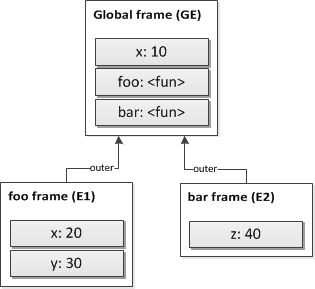##### 函数创建和执行规则

``// 全局 "x"``var x = 10;``// 函数 "foo" 相对于全局环境创建``function foo(y) {``  var z = 30;``  console.log(x + y + z);``}``

``// 创建函数 "foo"``foo = functionObject {``  code: "console.log(x + y + z);"``  environment: {x: 10, outer: null}``};``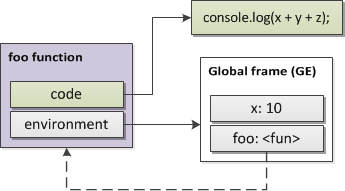``// 函数 "foo" 以参数 20 调用``foo(20);``

``// 用形参和局部变量创建新的帧``fooFrame = {``  y: 20,``  z: 30,``  outer: foo.environment``};``// 执行函数 "foo" 的代码``execute(foo.code, fooFrame); // 60``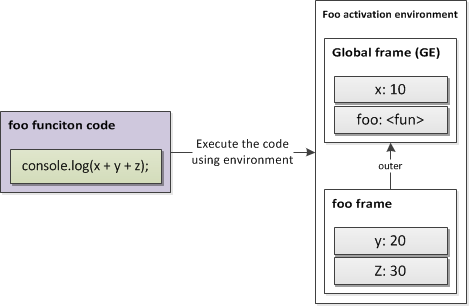##### 函数参数问题

``(function (x) {``  return function (y) {``    return x + y;``  };``})(10)(20); // 30``

``var x = 10;``(function (funArg) {``  var x = 20;``  funArg(); // 10，而不是 20``})(function () { // 创建并传递一个函数``  console.log(x);``});``

#### 组合的环境帧模型

``// 全局环境``var x = 10;``var y = 20;``function foo(z) {``  // 函数 "foo" 的环境``  var q = 40;``  function bar() {``    // 函数 "bar" 的环境``    return x + z;``  }``  return bar;``}``// 创建 "bar"``var bar = foo(30);``// 创建 "bar"``bar();``

``bar = closure {``  code: <...>,``  environment: {``    x: 10,``    z: 30,``  }``}``

``# Python 环境示例``# 全局 "x"``x = 10``# 全局函数 "foo"``def foo(y):``    # 局部 "z"``    z = 40``    # 局部函数 "bar"``    def bar():``        return x + y``    return bar``# 创建 "bar"``bar = foo(20)``# 执行 "bar"``bar() # 30``# 函数 "bar" 保存的环境；``# 存储在其 __closure__ 属性中；``#``# 只包含 {"y": 20}；``# "x" 不在 __closure__ 中，因为它``# 始终可以在全局被找到；``# "z" 也没有被保存，因为没有用到``barEnvironment = bar.__closure__``print(barEnvironment) # 闭包单元组成的元组``internalY = barEnvironment.cell_contents``print(internalY) # 20, "y"``

``def foo(x):``    def bar(y):``        print(eval(k))``    def baz(y):``        z = x``        print(eval(k))``    return [bar, baz]``# 创建函数 "bar" 和 "baz"``[bar, baz] = foo(10)``# "bar" 不包含任何闭包数据``print(bar.__closure__) # None``# "baz" 包含变量 "x"``print(baz.__closure__) # closure cells {'x': 10}``k = "y"``baz(20) # OK，20``bar(20) # OK，20``k = "x"``baz(20) # OK，10 - "x"``bar(20), # 错误，"x" 未定义``

``function foo(x) {``  function bar(y) {``    console.log(eval(k));``  }``  return bar;``}``// 创建 "bar"``var bar = foo(10);``var k = "y";``// 执行 bar``bar(20); // OK，20 - "y"``k = "x";``bar(20); // OK，10 - "x"``

Ruby 语言也采用了单个帧，捕获所有在闭包创建时存在的变量。下面例子中，Ruby 变量 `x` 被第二个闭包捕获，但第一个没有捕获：

``# Ruby lambda 闭包示例``# 闭包 "foo"，包含自由变量 "x"``foo = lambda {``  print x``}``# 定义 "x"``x = 10``# 第二个闭包 "bar" 有相同的函数体``# 也引用了自由变量 "x"``bar = lambda {``  print x``}``bar.call # OK，10``foo.call # 错误，"x" 未定义``

``k = "y"``foo = lambda { |x|``  lambda { |y|``    eval(k)``  }``}``# 创建 "bar"``bar = foo.call(10)``print(bar.call(20)) # OK，20 - "y"``k = "x"``print(bar.call(20)) # OK, 10 - "x"``

``-- Lua 环境示例：``-- 全局 "x"``x = 10``-- 全局函数 "foo"``function foo(y)``  -- 局部变量 "q"``  local q = 40``  -- 获取 "foo" 的环境``  fooEnvironment = getfenv(foo)``  -- {x = 10, globals...}``  print(fooEnvironment) -- table``  -- "x" 和 "y" 可以被获取，``  -- 因为 "x" 在环境中，而``  -- "y" 是局部变量（参数）``  print(x + y) -- 30``  -- 现在改变 "foo" 的环境``  setfenv(foo, {``     -- 引用 "print" 函数，``     -- 给出另外的名称``    printValue = print,``    -- 重用 "x"``    x = x,``    -- 定义一个新的绑定 "z"``    -- 值为 "y"``    z = y``  })``  -- 使用新的绑定``  printValue(x) -- OK，10``  printValue(x + z) -- OK，30``  -- 局部变量仍然可以访问``  printValue(y, q) -- 20，40``  -- 不过其他的名称``  printValue(print) -- nil``  print("test") -- 错误，"print" 名称是 nil，不能调用``end``foo(20)``

## 结论

• 环境的概念对应作用域的概念。

• 理论上有两种作用域：动态和静态。

• ECMAScript 使用静态（词法）作用域。

• 不同， `with``eval` 可以认为是给静态作用域带来动态特性。

• 作用域、环境、激活绝对性、激活记录、调用栈帧、环境帧、环境记录和执行上下文的概念，是近似的，并且应用在讨论中。所以，技术上来讲，在 ECMAScript 中，它们是彼此的一部分。例如，环境记录时词法环境的一部分，词法环境又是执行上下文的一部分。不过，逻辑上来说，它们可以近似相等。这些说法很正常：“全局作用域”、“全局环境”、“全局上下文”，等等。

• ECMAScript 使用链式的环境帧模型。在 ES3 中被称作作用域链。在 ES5 中环境帧被称作环境记录。

• 一个环境可以包含多个内部环境。

• 词法环境用于实现闭包和解决函数参数问题。

• ECMAScript 中的所有函数都是一等的，都是闭包。

• ECMA-262-5 详解 - 3.1 词法环境：通用理论 – ds.laboratory

这一节，我们会讨论词法环境的细节，它是在一些编程语言中用于管理静态作用域的一种机制。为了确保能充分理解这一主题，我们会简要讨论下其对立面：动态作用域（并没有直接用于 ECMAScript）。我们会看到环境是如何管理代码中的词法嵌套结构，以及为闭包提供全面支持。

发布：2018-11-03 阅读(2212)

• ECMA-262-5详述 第一章. 属性和属性描述符

这一章专门讨论了ECMA-262-5 规范的新概念之一 — 属性特性及其处理机制 — 属性描述符。 当我们说“一个对象有一些属性”的时候，通常指的是属性名和属性值之间的关联关系。但是，正如在ES3系列文章中分析的那样，一个属性不仅仅是一个字符串名，它还包括一系列特性—比如我们在ES3系列文章中已经讨论过的{ReadOnly}，{DontEnum}等。因此从这个观点来看，一个属性本身就是一个对象

发布：2018-10-26 阅读(1538)